# Tree Basics & Tricks

Updated:

⚠️ This post was created when I was in high school and is no longer maintained.

## 1. Tree Properties

1. The depth of a node is the number of edges from the node to the tree’s root node. A root node will have a depth of 0.

2. The height of a node is the number of edges on the longest path from the node to a leaf. A leaf node will have a height of 0.

3. The height of a tree is the height of the root or the maximal depth. (For a complete binary tree, its height is Θ(lgn) )

4. A level or layer of a tree consists of all nodes of the same depth the number of levels is the height of the tree + 1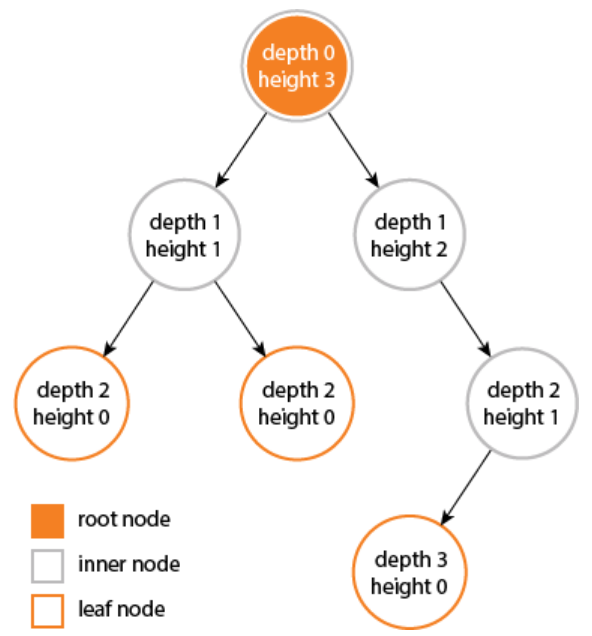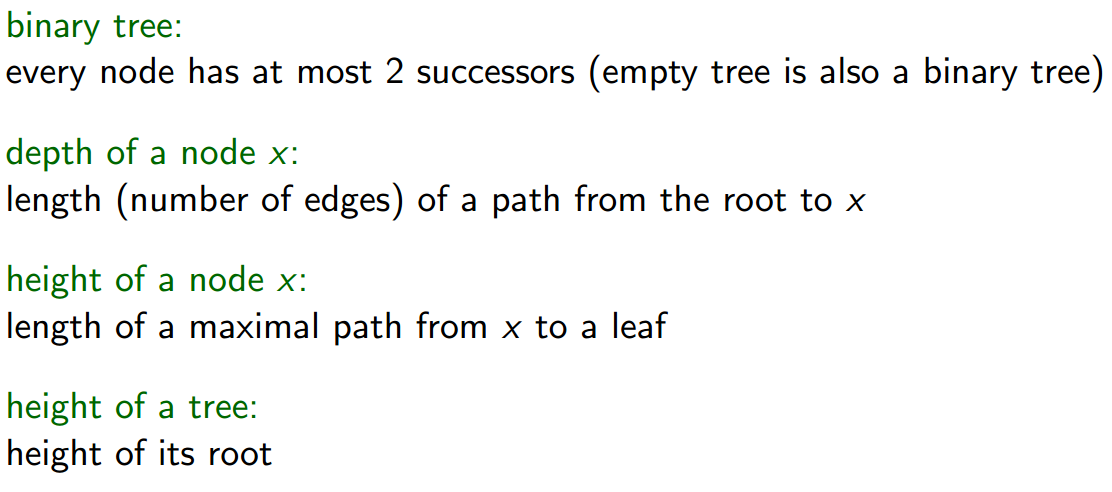## 2. Tree normal traversal

There are several ways to implement a Tree. The most common one is using a linked data structure in which, in addition to a key and satellite data each node has left, right and parents pointers.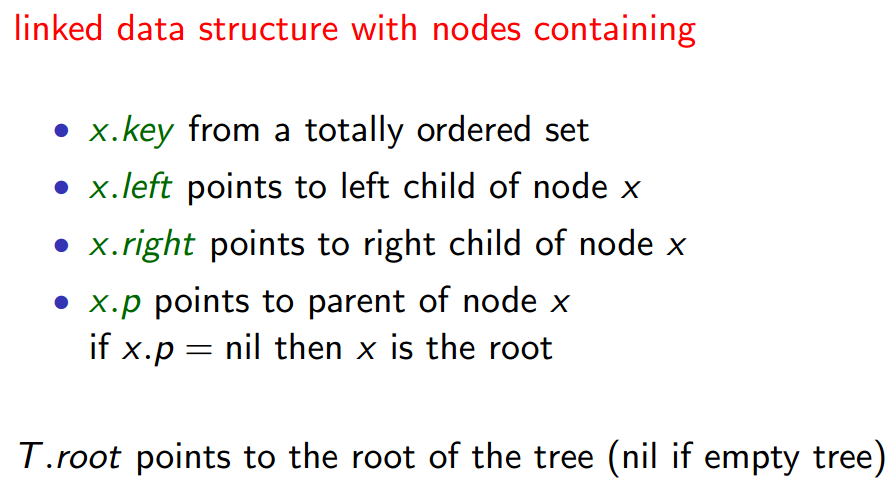<1> InOrder Traversal Visit left sub-tree, then node itself, then right sub-tree

algorithm InOrderTravesal(n):
if n != NIL then
InOrderTraversal(n.left)
-- only visit(print) itself when returning from the left sub-tree --
Visit(n)
InOrderTraversal(n.right)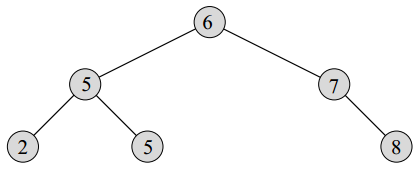Output: 2, 5, 5, 6, 7, 8

<2> PostOrder Traversal Visit all successors and next the node itself

algorithm PostOrderTraversal(n):
if m.left != NIL then
PostOrderTraversal(n.left)
if m.right != NIL then
PostOrderTraversal(n.right)
Visit(n)
-- only visit(print) itself when returning from both sub-tree --


Output: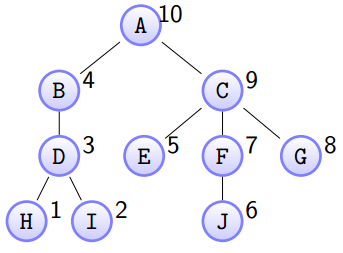(Numbers represent traversal order)

<3> PreOrder Traversal First visit itself and next its successors

algorithm PreOrderTraversal(n):
Visit(n)
if m.left != NIL then
PostOrderTraversal(n.left)
if m.right != NIL then
PostOrderTraversal(n.right)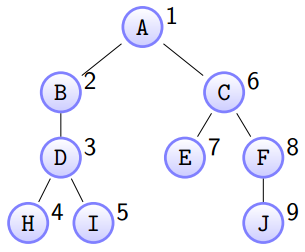Tip:

• In my point of view, the embedded (inside) recursion functions are just entries that represent execution order. The code below an entry will not be executed until that entry was bounced back (returned).
• the .left and .right for a leaf are both NIL so for inorder traversal, instead of if n != NIL then ..., we could do if n.left != NIL and n.right != NIL then ... for the sake of intuition.
• to better understand these traversal algorithms, bear in mind that the order of a given traversal method should be satisfied in every subtree.

## 4. Tree traversal augmentations (tricks)

There are some interesting implementations of traversing a tree structure I’d like to share:

1. Non-recursive preorder traversal:
Algorithm NonRecurPreOrder(startNode):
S := new Stack
Push(S, startNode)

While not S.isEmpty() do
node := Pop(S)

While node != NIL do:
Visit(node)
Push(S, node.right)
node := node.left

1. Non-recursuve inorder traversal:
Algorithm NonRecurInOrder(startNode):
if startNode == NIL then return

S := new Stack
Push(S, startNode)
node := startNode

While not S.isEmpty() do
While node.left != NIL do
node := node.left
Push(S, node)

node := Pop(S)
Visit(node)
-- the most beatiful logic is here --
if node.right != NIL then
node := node.right
Push(S, node)



## 5. InOrder Traversal Time Complexcity

$T(n) = Θ(n)$ Proof: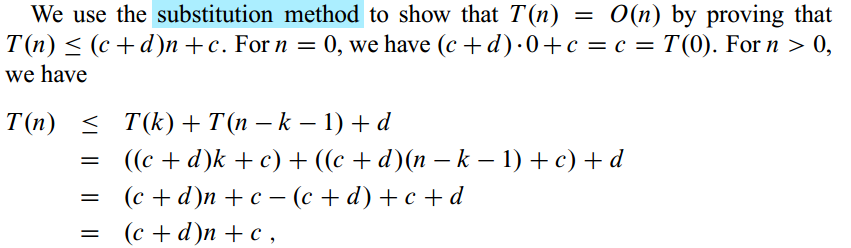## 6. Today’s special

###Reserve singly linked-list non-recursively in Θ(n)

Algorithm ReserveSLL(L):
return

prevPtr.next := NIL     --> After revserving, L.head will becomes the new tail whose next should be NIL
nextPtr := NIL

While currPtr != NIL do
nextPtr := currPtr.next
currPtr.next := prePtr
prevPtr := currPtr
currPtr := nextPtr

L.head := prevPtr --> Don't forget to set the head in the end


Tags: